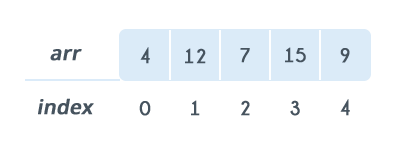Data Structures
Topics:
1-D
• Arrays
• Stacks
• Queues
• Hash Tables
• Trees
• Disjoint Data Structures

# 1-D

An array is a sequential collection of elements of same data type and stores data elements in a continuous memory location. The elements of an array are accessed by using an index. The index of an array of size N can range from $0$ to $N−1$. For example, if your array size is $5$, then your index will range from 0 to 4 (5-1). Each element of an array can be accessed by using $arr[index]$.

Consider following array. The size of this array is $5$. If you want to access $12$, then you can access it by using arr[ 1 ] i.e. $12$.Array declaration

Declaring an array is language-specific.

For example, in C/C++, to declare an array, you must specify, the following:

• Size of the array: This defines the number of elements that can be stored in the array.

• Type of array: This defines the type of each element i.e. number, character, or any other data type.

A C++ example would be:

int arr;


This is a static array and the other kind is dynamic array, where type is just enough for declaration. In dynamic arrays, size increases as more elements are added to the array.

Array Initialization:

Array can be initialized either at the time of declaration or after that.

The sample format if an array is initialized at the time of declaration is

type arr[size] = {elements}


The sample format of an array that is initialized in C++, is

int arr = {4, 12, 7, 15, 9};


An array can be initialized after declaration by assigning values to each index of the array as follows

 type arr[size]
arr[index] = 12


C++ example:

int arr;
arr = 4;
arr = 12;


Processing an Array:

The most basic form of processing an array is to loop over the array and print all its elements.

A sample of processing an array by looping over the array and printing its elements is as follows:

type arr[size] = {elements}
for idx from 0 to size
print arr[idx]


C++ example:

#include <iostream>
using namespace std;

int main()
{
// Array declaration and initialization
int arr = {4, 12, 7, 15, 9};
// Iterate over the array
for(int idx=0; idx<5; idx++)
{
// Print out each element in a new line
cout << arr[idx] << endl;
}
return 0;
}


A practice problem is be a great way to apply these concepts. Try solving one here!

Contributed by: Ravi Ojha